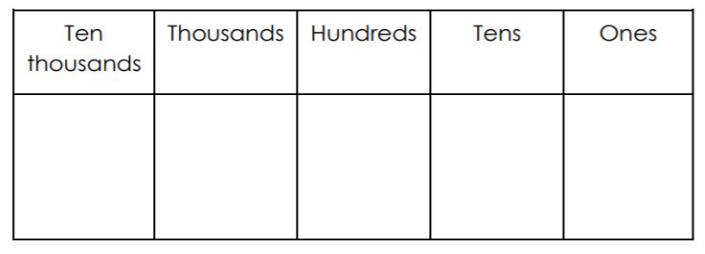Roll the dice 5 times.

Each time you roll, decide where to place your number on the place value chart to make the number with the greatest value.

Explain your reasoning.

Repeat this process 5 times.

I have done one to show you an example.Explanation:

I put the 6 in the ten thousands column because it is the highest value column and 6 is the biggest digit. 5 is the next biggest number so I put it in the thousands column. I put the 2 and the 2 in the tens and ones because they are the smallest numbers and these columns are the least in value.Explanation:

Explanation

ExplanationExplanation

﻿